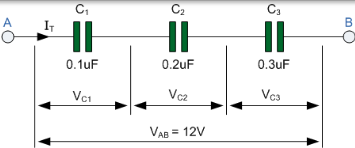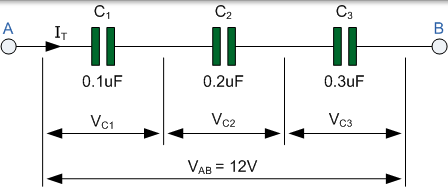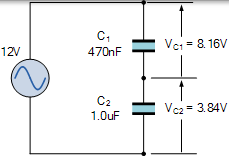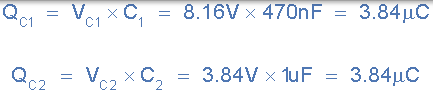# 串联电容器QT = Q1 = Q2 = Q3 …等

### 串联连接中的电容器$$V_{AB}=V_{C1}+V_{C2}+V_{C3} = 12V$$
$$V_{C1} = {Q_{T}\over C_{1}}, \quad V_{C2} = {Q_{T}\over C_{2}}, \quad V_{C2} = {Q_{T}\over C_{3}}$$

$$V_{AB}={Q_{T}\over C_{T}} = {Q_{T}\over C_{1}}+{Q_{T}\over C_{2}}+{Q_{T}\over C_{3}}$$

### 串联电容器方程

$${1\over C_{T}} = {1\over C_{1}}+{1\over C_{2}}+{1\over C_{3}}+...+...$$

## 电容器串联示例 No1

$${1\over C_{T}} = {1 \over 0.1\mu F}+{1 \over 0.2\mu F}+{1 \over 0.3\mu F}={1 \over 18.33 \times 10^{6}}$$

$$C_{T} = {C_{1} \times C_{2} \over C_{1} + C_{2}}$$

$$C_{T} = {C^{2} \over C_{1} + C_{2}} = {C \over 2} = {1 \over 2} C$$

$$V_{CX}=V_{S} {C_{T} \over C_{X}}$$

## 电容器串联示例 No2

• a） 两个电容器，每个电容器的电容为 47nF
• b） 一个 470nF 的电容串联连接到 1μF 的电容

a） 总等容电容，

$$C_{T} = {C_{1} \times C_{2} \over C_{1} + C_{2}} = {47nF \times 47nF \over 47nF + 47nF}=23.5nF$$

$$V_{C1} = {C_{T} \over C_{1}} = {23.5nF \over 47nF} \times 12 V = 6 Volts$$
$$V_{C2} = {C_{T} \over C_{2}} = {23.5nF \over 47nF} \times 12 V = 6 Volts$$

b） 不相等电容，

$$C_{T} = {C_{1} \times C_{2} \over C_{1} + C_{2}} = {470nF \times 1 \mu F \over 470nF + 1 \mu F}=230nF$$

$$V_{C1} = {C_{T} \over C_{1}} = {230nF \over 470nF} \times 12 V = 8.16 Volts$$
$$V_{C2} = {C_{T} \over C_{2}} = {230nF \over 1 \mu F} \times 12 V = 3.84 Volts$$